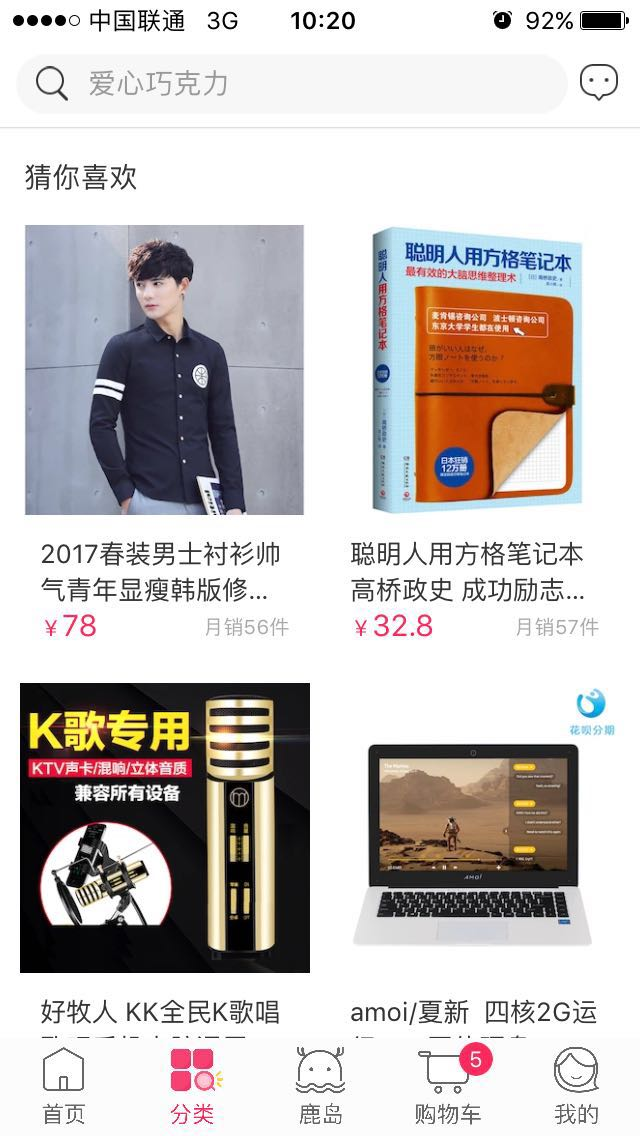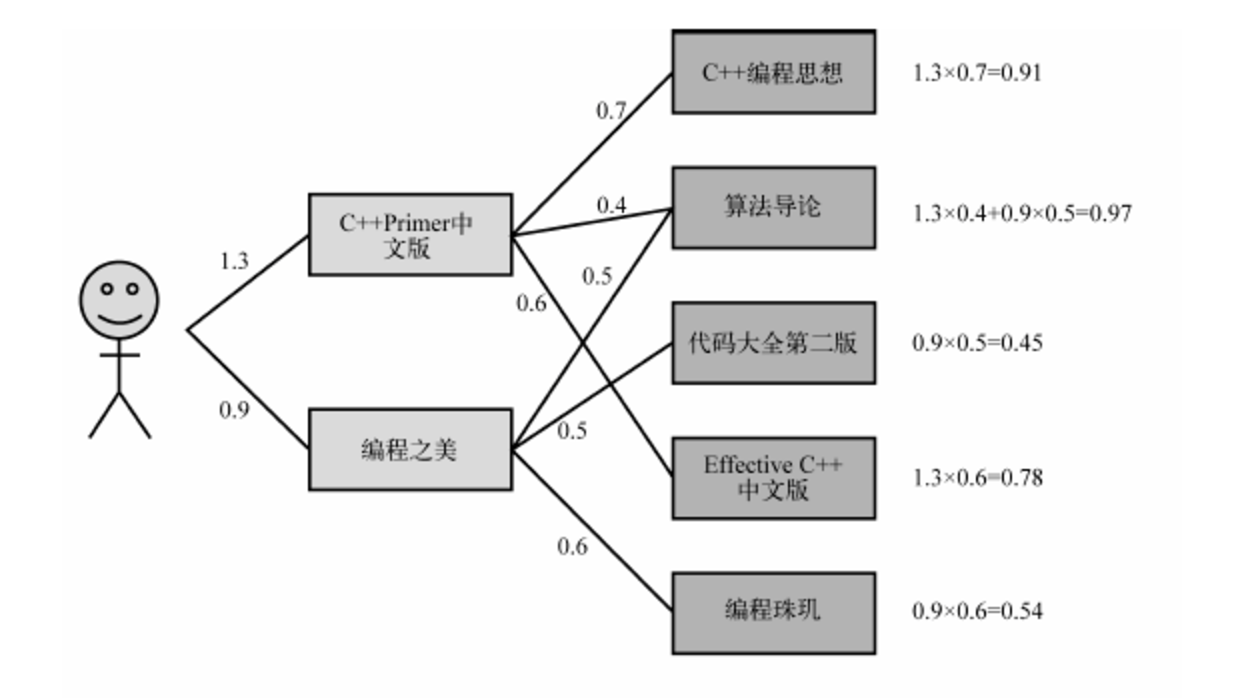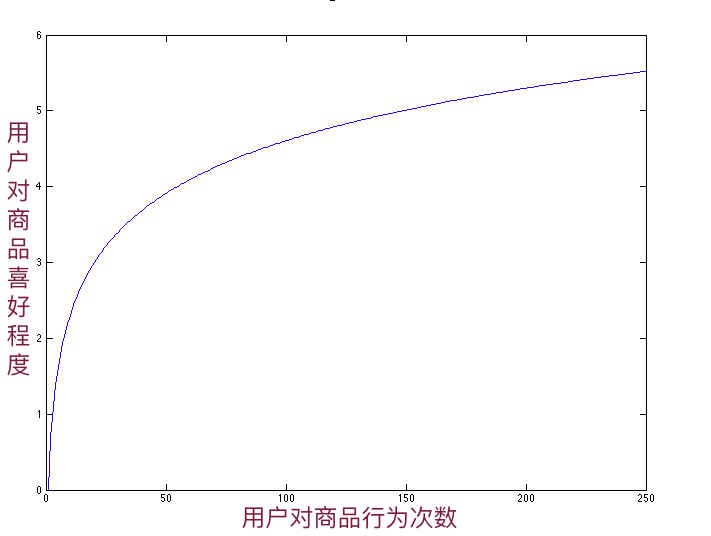## 问题背景## 协同过滤

• 基于用户的协同过滤算法（user-based collaboratIve filtering）
• 基于物品的协同过滤算法（item-based collaborative filtering）### Jaccard相似系数

Jaccard 相似系数是衡量两个集合相似度的一种指标，公式如下：

$$sim_{Jaccard}(a, b) = \frac{|A \cap B|}{|A \cup B|}.$$

### 余弦相似系数

$$sim_{cosine}(a, b) = \frac{\vec{A}\cdot\vec{B}}{\Vert A \Vert \cdot \Vert B \Vert}.$$

• $(A_{1}, A_{2}, \cdots, A_{n})$
• $(B_{1}, B_{2}, \cdots, B_{m})$

$$sim_{cosine}(a, b) = \frac{\sum_{i=1}^{k}A_{i}\cdot B_{i}}{\sqrt{A_{1}^{2}+A_{2}^{2}+\cdots+A_{n}^{2}}\cdot \sqrt{B_{1}^{2}+B_{2}^{2}+\cdots+B_{m}^{2}}}.$$

### 皮尔逊相关系数

$$\begin{eqnarray} \rho_{a,b} & = & \frac{cov(A,B)}{\sigma_{A}\cdot\sigma_{B}} \\ {} & = & \frac{E((A-\mu_{A})\cdot(B-\mu_{B}))}{\sigma_{X}\cdot\sigma_{B}} \\ {} & = & \frac{E(A\cdot B)-E(A)\cdot E(B)}{\sqrt{E(A^{2})-E^{2}(A)}\cdot\sqrt{E(B^{2})-E^{2}(B)}}. \end{eqnarray}$$

### 欧几里德距离

$$sim_{Euclid}(a, b) = \sqrt{(A_{1}-B_{1})^{2}+(A_{2}-B_{2})^{2}+\cdots+(A_{n}-B_{n})^{2}}.$$

## 个性化推荐

1. 基于所有用户对商品的历史行为，计算出每个商品的相似商品；
2. 对每个用户有过直接行为的商品，寻找出相似的商品推荐给用户。

### 寻找相似商品

$score=(s_{click}, s_{fav}, s_{cart}, s_{order}).$

$score(U, I) = \sum_{b \in \{click, fav, cart, order\}}(w_{b}\cdot s_{b}).$

$$w_{b}=\begin{cases} 1 & \text{count(b)>0},\\ 0 & \text{count(b)=0}. \end{cases}$$

$count(b)$，$b \in \{click, fav, cart, order\}$，分别代表4种行为的次数。

• $((I_{a}, U_{a, 1}, score(I_{a}, U_{a, 1}), (I_{a}, U_{a, 2}, score(I_{a}, U_{a, 2}),\cdots, (I_{a}, U_{a, n}, score(I_{a}, U_{a, n}))$
• $((I_{b}, U_{b, 1}, score(I_{b}, U_{b, 1}), (I_{b}, U_{b, 2}, score(I_{b}, U_{b, 2}),\cdots, (I_{b}, U_{b, m}, score(I_{b}, U_{b, m}))$

$$\begin{eqnarray} sim_{cosine}(a, b) = \frac{\sum_{k=1}^{K}(score(I_{a}, U_{a,k})\cdot score(I_{b}, U_{b,k}))}{\sqrt{\sum_{i=1}^{n}score^{2}(I_{a}, U_{a, i})}\cdot\sqrt{\sum_{j=1}^{m}score^{2}(I_{b}, U_{b, j})}}. \end{eqnarray}$$

### 寻找用户喜欢商品• $(I_{1}, I_{2}, \cdots, I_{n})$

• $(I_{i,1}, I_{i, 2}, \cdots, I_{i, m})$

$$\begin{eqnarray} like(U, I)=\sum_{i=1}^{n}(score(I_{i}, U)\cdot sim_{cosine}(I_{i}, I)). \end{eqnarray}$$

## 算法优化

### 基于业务背景优化

#### 活跃用户

1. 活跃用户行为不纳入计算商品相似度的数据集范围中；
2. 活跃用户行为小于相对不活跃用户行为对商品相似度的贡献，活跃用户对商品的评价降权，一种降权的算法如下

$$w^{u} = \frac{log2.0}{log(3.0+count_{U}(I))}.$$

• 活动商品

• 虚拟商品

#### 用户行为的权重、主动性、频率

• 用户行为权重

$score=(s_{click}, s_{fav}, s_{cart}, s_{order}).$

• 用户行为主动性

• 用户行为的频率#### 时间衰减

$score_{click, n}(U, I)=\alpha^{n}\cdot score_{click}(U, I).$

$score_{click, 1}(U, I)=\alpha^{1}\cdot score_{click}(U, I).$

## 模型问题

### 用户冷启动

1. 基于用户实时的CLICK、FAV、CART和ORDER行为，寻找这些行为对应的商品的相似商品推荐给用户；
2. 基于用户的特征，如性别、地域、手机操作系统(IOS/Android)、手机品牌(如vivo、apple、xiaomi)，统计不同特征下的热销商品，组合推荐给用户。

## 参考资料

1. 项亮，《推荐系统实践》，人民邮电出版社
2. Spark性能优化指南——基础篇
3. 余弦距离、欧氏距离和杰卡德相似性度量的对比分析
4. Spark对数据倾斜的八种处理方法